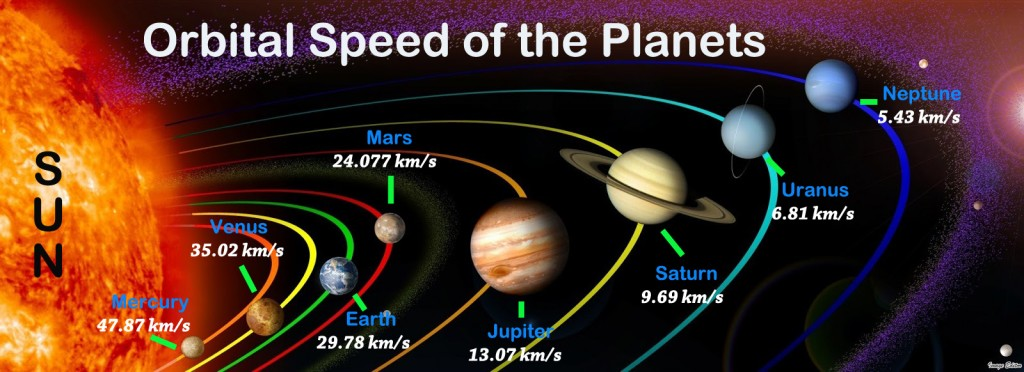The orbital speeds of the planets vary depending on their distance from the sun. This is because of the gravitational force being exerted on the planets by the sun. Additionally, according to Kepler’s laws of planetary motion, the flight path of every planet is in the shape of an ellipse. Below is a list of the planet’s orbital speeds in order from fastest to slowest.1. Mercury is the fastest planet, which speeds around the sun at 47.87 km/s. In miles per hour this equates to a whopping 107,082 miles per hour.

2. Venus is the second fastest planet with an orbital speed of 35.02 km/s, or 78,337 miles per hour.

3. Earth, our home planet of Earth speeds around the sun at a rate of 29.78 km/s. This means that we are traveling at 66,615 miles per hour.

4. Mars, with an orbital speed of 24.077 km/s, or 53,858 miles per hour, travels considerably faster than the prior planets.

5. Jupiter travels a bit faster than the previous three planets with an orbital speed of 13.07 km/s. This translates to approximately 29,236 miles per hour.

6. Saturn travels at 9.69 km/s, or 21,675 miles per hour, which makes it the third slowest planet.

7. Uranus is the second slowest planet with an orbital speed of 6.81 km/s. This equates to 15,233 miles per hour.

8. Neptune travels around the sun at a speed of 5.43 km/s or 12,146 miles per hour. Although this is a very high rate of speed, Neptune still has the slowest orbital velocity of any of the planets.